# Fillna in multiple columns in place in Python Pandas

In this article, we are going to write Python script to fill multiple columns in place in Python using pandas library. A data frame is a 2D data structure that can be stored in CSV, Excel, .dB, SQL formats. We will be using Pandas Library of python to fill the missing values in Data Frame.

## Fillna in multiple columns inplace

First creating a Dataset with pandas in Python

## Python3

 `# Importing Required Libraries` `import` `pandas as pd` `import` `numpy as np`   `# Creating a sample dataframe with NaN values` `dataframe ``=` `pd.DataFrame({``'Count'``: [``1``, np.nan,` `                                    ``np.nan, ``4``,` `                                    ``2``, np.nan,` `                                    ``np.nan, ``5``, ``6``],` `                          `  `    ``'Name'``: [``'Geeks'``,``'for'``, ``'Geeks'``,``'a'``,``'portal'``,``'for'``,` `             ``'computer'``, ``'Science'``,``'Geeks'``],` `                          ``'Category'``:``list``(``'ppqqrrsss'``)})`   `# Printing The dataframe` `display(dataframe)`

Output: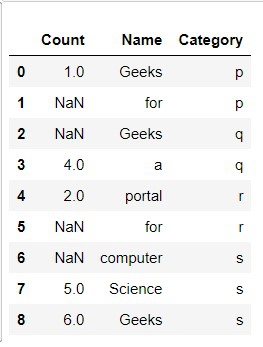Fillna in multiple columns in place

### Example 1: Filling missing columns values with fixed values:

We can use fillna() function to impute the missing values of a data frame to every column defined by a dictionary of values. The limitation of this method is that we can only use constant values to be filled.

## Python3

 `# Importing Required Libraries` `import` `pandas as pd` `import` `numpy as np`   `# Creating a sample dataframe with NaN values` `dataframe ``=` `pd.DataFrame({``'Count'``: [``1``, np.nan, np.nan, ``4``, ``2``,` `                                    ``np.nan,np.nan, ``5``, ``6``], ` `                          `  `    ``'Name'``: [``'Geeks'``,``'for'``, ``'Geeks'``,``'a'``,``'portal'``,``'for'``,` `             ``'computer'``, ``'Science'``,``'Geeks'``],` `                          ``'Category'``:``list``(``'ppqqrrsss'``)})`   `# Creating a constant value for column Count` `constant_values ``=` `{``'Count'``: ``10``}` `dataframe ``=` `dataframe.fillna(value ``=` `constant_values)`   `# Printing the dataframe` `display(dataframe)`

Output: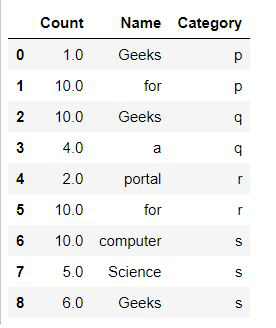Fillna in multiple columns in place

### Example 2: Filling missing columns values with mean():

In this method, the values are defined by a method called mean() which finds out the mean of existing values of the given column and then imputes the mean values in each of the missing (NaN) values.

## Python3

 `# Importing Required Libraries` `import` `pandas as pd` `import` `numpy as np`   `# Creating a sample dataframe with NaN values` `dataframe ``=` `pd.DataFrame({``'Count'``: [``1``, np.nan, np.nan, ``4``, ``2``,` `                                    ``np.nan,np.nan, ``5``, ``6``], ` `                          `  `    ``'Name'``: [``'Geeks'``,``'for'``, ``'Geeks'``,``'a'``,``'portal'``,``'for'``,` `             ``'computer'``, ``'Science'``,``'Geeks'``],` `                          ``'Category'``:``list``(``'ppqqrrsss'``)})`     `# Filling Count column with mean of Count column` `dataframe.fillna(dataframe[``'Count'``].mean(), inplace ``=` `True``)`   `# Printing the Dataframe` `display(dataframe)`

Output: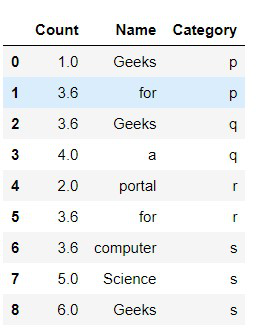Fillna in multiple columns in place

### Example 3: Filling missing column values with mode().

The mode is the value that appears most often in a set of data values. If X is a discrete random variable, the mode is the value x at which the probability mass function takes its maximum value. In other words, it is the value that is most likely to be sampled.

## Python3

 `# Importing Required Libraries` `import` `pandas as pd` `import` `numpy as np`   `# Creating a sample dataframe with NaN values` `dataframe ``=` `pd.DataFrame({``'Count'``: [``1``, np.nan, np.nan,` `                                    ``1``, ``2``, np.nan,np.nan,` `                                    ``5``, ``1``], ` `                          `  `    ``'Name'``: [``'Geeks'``,``'for'``, ``'Geeks'``,``'a'``,``'portal'``,``'for'``,` `             ``'computer'``, ``'Science'``,``'Geeks'``],` `                          ``'Category'``:``list``(``'ppqqrrsss'``)})`   `# Using Mode() function to impute the values using fillna` `dataframe.fillna(dataframe[``'Count'``].mode()[``0``], inplace ``=` `True``)`   `# Printing the Dataframe` `display(dataframe)`

Output: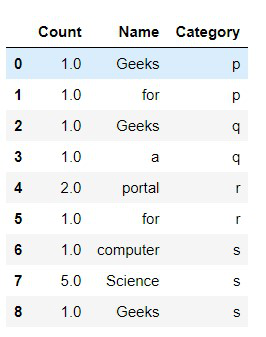Fillna in multiple columns in place

### Example 4: Filling missing column values with multiple values.

Here we are filling the multiple values in the missing columns with the defined values.

## Python3

 `# Importing Required Libraries` `import` `pandas as pd` `import` `numpy as np`   `# Creating a sample dataframe with NaN values` `dataframe ``=` `pd.DataFrame({``'Count'``: [``1``, np.nan,` `                                    ``np.nan, ``4``,` `                                    ``2``, np.nan,` `                                    ``np.nan, ``5``, ``6``],` `                          `  `    ``'Name'``: [``'Geeks'``,``'for'``, np.nan,``'a'``,``'portal'``,``'for'``,` `             ``'computer'``, np.nan,``'Geeks'``],` `                          ``'Category'``:``list``(``'ppqqrrsss'``)})` `dataframe.fillna({``'Count'``:``'Unknown'``, ``'Name'``: ``'GFG'``}, inplace``=``True``)`   `#view DataFrame` `display(dataframe)`

Output: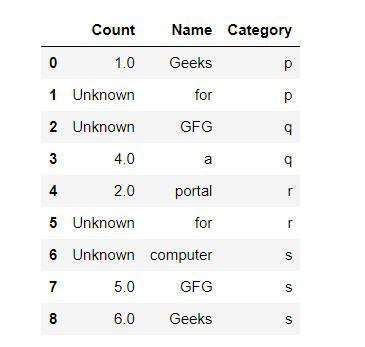Fillna in multiple columns in place

Whether you're preparing for your first job interview or aiming to upskill in this ever-evolving tech landscape, GeeksforGeeks Courses are your key to success. We provide top-quality content at affordable prices, all geared towards accelerating your growth in a time-bound manner. Join the millions we've already empowered, and we're here to do the same for you. Don't miss out - check it out now!

Previous
Next